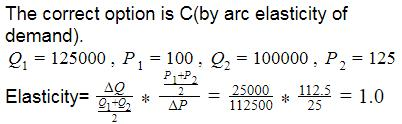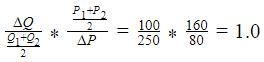Courses

# Test: Theory Of Demand- 3

## 30 Questions MCQ Test Business Economics for CA Foundation | Test: Theory Of Demand- 3

Description
This mock test of Test: Theory Of Demand- 3 for CA Foundation helps you for every CA Foundation entrance exam. This contains 30 Multiple Choice Questions for CA Foundation Test: Theory Of Demand- 3 (mcq) to study with solutions a complete question bank. The solved questions answers in this Test: Theory Of Demand- 3 quiz give you a good mix of easy questions and tough questions. CA Foundation students definitely take this Test: Theory Of Demand- 3 exercise for a better result in the exam. You can find other Test: Theory Of Demand- 3 extra questions, long questions & short questions for CA Foundation on EduRev as well by searching above.
QUESTION: 1

Solution:
QUESTION: 2

Solution:
QUESTION: 3

### Which of the following is not determinant of demand?

Solution:
QUESTION: 4

Cross elasticity of complementary goods is:

Solution:
QUESTION: 5

Generally, when income of consumer increases, he goes in for superior goods, leading to a fall in demand for inferior goods. It means, income elasticity of demand is ________.

Solution:
QUESTION: 6

In which of the following cases the demand for goods tends to be less elastic?

Solution:
QUESTION: 7

Demand for electricity power is elastic because_______

Solution:
QUESTION: 8

Bricks for houses is an example of which kind of demand?

Solution:
QUESTION: 9

What will be the price elasticity it original price is Rs. 5, original quantity is 8 units and changed price is Rs. 6, changed quantity is 4 units:

Solution:
QUESTION: 10

In expansion and contraction of demand _____

Solution:
QUESTION: 11

The price of a commodity decreases from 10 to 8 and the quantity demanded of it increases from 25 to 30 units, then the coefficient of price elasticity will be______.

Solution:
QUESTION: 12

What is the elasticity between mid-point and upper extreme point of a straight line continuous demand curve?

Solution:
QUESTION: 13

The demand for factors of production is ______.

Solution:
QUESTION: 14

Expansion and contraction in demand are caused by:

Solution:
QUESTION: 15

A 10% increase in the price of tea results is an 8% increase in the demand for coffee. Cross elasticity of demand will be:

Solution:
QUESTION: 16

In case of luxury goods, the income elasticity of demand will be_______

Solution:
QUESTION: 17

Increase in Price from Rs. 4 to Rs. 6 then decrease in demand from 15 units to 10 units. What is the price elasticity. (Point elasticity)

Solution:
QUESTION: 18

In case of a straight line demand curve meeting the two axes, the price elasticity of demand at the mid-point of the line would be:

Solution:
QUESTION: 19

Price of Tiffin Box is Rs. 100 per unit and the quantity demanded in market is 1,25,000 units. Company increased the price to Rs. 125. Due to this increase in price, quantity demanded decreases to 1, 00,000 units. What will be the price elasticity of demand?

Solution:QUESTION: 20

In case of an inferior good, the income elasticity of demand is:

Solution:
QUESTION: 21

Cross elasticity of complementary goods is:

Solution:
QUESTION: 22

Which of the following elasticity of demand measures a movement along the demand curve rather than a shift in the curve?

Solution:
QUESTION: 23

Generally, when income of consumer increases, he goes in for superior goods, leading to a fall in demand for inferior goods. It means, income elasticity of demand is ________.

Solution:
QUESTION: 24

For a commodity with a unitary elastic demand curve if the price of the commodity raises, then the consumer’s total expenditure on this commodity would:

Solution:
QUESTION: 25

Suppose the price of movies seen at a theatre rises form Rs. 120 per person to Rs.200 per person. The theatre manager observed that the rise in prices has lead to a fall in attendance at a given movie from 300 persons to 200 persons. What is the price elasticity of demand for the movie? (arc elasticity)

Solution:

The correct option is B(by arc elasticity)

Q1=300 , P1=120 , Q2=200 , P2=200

Elasticity =QUESTION: 26

A consumer spends Rs. 80 on purchasing a commodity when its price is Re. 1 per unit and spends Rs. 96 when the price is Rs. 2 per unit. Calculate the price elasticity of demand.

Solution:
QUESTION: 27

When price falls from Rs. 6 to Rs. 4, the demand rises form 10 to 15 units. Calculate price elasticity of demand. (Point elasticity)

Solution:
QUESTION: 28

The price of hot-dogs increases by 22% and the quantity demanded falls by 25% this indicates that demand for hot dogs is:

Solution:
QUESTION: 29

A 10% increase in the price of tea results is an 8% increase in the demand for coffee. Cross elasticity of demand will be:

Solution:
QUESTION: 30

What is income elasticity of demand, when income changes by 20% and demand changes by 40%

Solution: